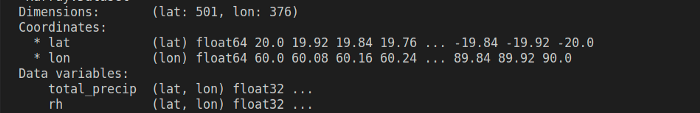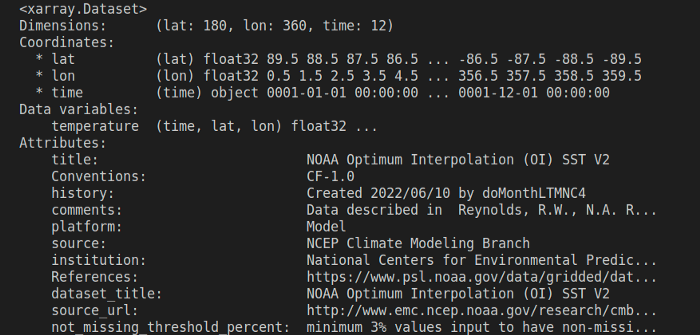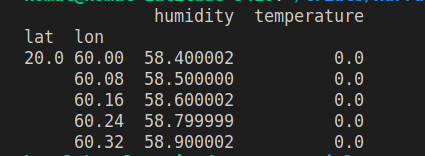# Introduction to XarrayKomal Tarte

November 07, 2022 | 5 minutes read

Are you a Python developer, who is head over heels for Pandas? Of Course, you are!

NetCDF (Network Common Data Form) is a set of software libraries and self-describing, machine-independent data formats that support the creation, access, and sharing of array-oriented scientific data.

• Dimensions: these are used to specify the shape of one or more of the multi-dimensional variables contained in a NetCDF file. You can think of them as physical quantities. For eg. latitude, longitude, depth, time, altitude etc.
• Data Variables: These represent an array of values of the same type. They have a name, shape, and a datatype associated with them eg, sea surface temperature, precipitation, air pressure etc.
• Coordinate Variables: These are variables with the same name as that of the dimensions. They define a physical coordinate corresponding to that dimension.
• Attributes: NetCDF attributes store information about the data.When I started dealing with NC files, which are datasets containing n-dimensions, variables, and attributes, I could no longer rely on Pandas. Because when your data starts getting complex with N-dimensions, and you can no longer classify it into simple rows and columns, Pandas be like,1. DataArrays that wrap underlying data containers (e.g. NumPy arrays) and contain associated metadata.
2. DataSets that are dictionary-like containers of DataArrays. It is very similar to the pandas’ data frame.
• Python (3.8 or later)
• numpy (1.20 or later)
• pandas (1.3 or later)
• netcdf4 (Xarray needs this engine to deal with NetCDF files)

## Importing a NetCDF file

``` ```
import xarray as xr
try:
with xr.open_dataset('./temperature.nc') as ds:
print(ds)
except Exception as err:
print('oops...', err)
```
```You can extract data from a particular variable simply using the dot operator. ds.<data_array_name>

``` ```
ds.lat
```
```You can also query the dataset, using where()

``` ```
ds.where(ds.temperature < -1)
```
```

E.g. To convert any Xarray dataset to a Pandas DataFrame, you can use

``` ```
ds.to_dataframe()
```
```

Once you have a DataFrame you can apply any methods from pandas on it to get different views on the data

## Dealing with Multiple datasets

Here’s how you can open multiple datasets at once and convert them to a DataFrame.

``` ```
files_to_collate = ['temperature.nc', 'humidity.nc']
filters = 'temperature <= 0 & humidity > 50'
with xr.open_mfdataset(files_to_collate) as ds:
df = ds.to_dataframe().dropna(how="all")
filtered_df = df[df.eval(filters)]
print(filtered_df)
```
```

The eval() function evaluates a string describing operations on Pandas DataFrame columns. The resulting DataFrame has columns from both the dataset variables, mapped against the coordinates variables. Smooth right?